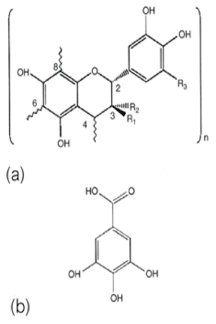Figure 1: (a) Grape proanthocyanidins: n, degree of polymerization. At the C4-C6 or C4-C8, the linkage of another molecule : (+)-catechin, (R1 = OH, R2 = H, R3 = H): (-)-epicatechin, (R1 = H, R2 = OH, R3 = H): (-)Epicatechin-3-o-gallate (R1=H, R2=O-galloyl, R3=H): (-)-Epigallocatechin: (R1=H, R2=OH, R3=OH) (b) The molecular structure of Gallic acid